Power query is a very powerful data extraction and transformation tool that comes baked into Excel 2016 (or later), Excel for Office 365 and Power BI.

It can be found in the Data tab in the Get & Transform Data section of the ribbon.

It’s very powerful and also very easy to use and the query editor has a super intuitive user interface for an Excel user. Many transformation steps can be easily performed from the power query editor ribbon and you don’t need to know any code to clean and shape your data.

Behind the scenes of the user friendly editor, Excel is translating each step in your transformation process from those ribbon commands into the power query M code language.

This post will introduce you to the basics of the power query M code language and will assume you already know the basics of power query.

## What Is M Code?

The M stands for data Mash-up, as power query is all about connecting to various different data sources and “Mashing” them up.

M code is the language behind the scenes of power query. When you create a data transformation in the power query editor UI, Excel is writing the corresponding M code for the query.

M is a functional language which means it is primarily written with functions that are called to evaluate and return results. M code comes with a very large library of predefined functions available and you can also create your own.

## Where Can You Write Power Query M Code?

If you want to start writing or editing M code, you’re going to need to know where you can do this. There are two places where it’s possible, in the formula bar or the advanced editor.

### The Formula Bar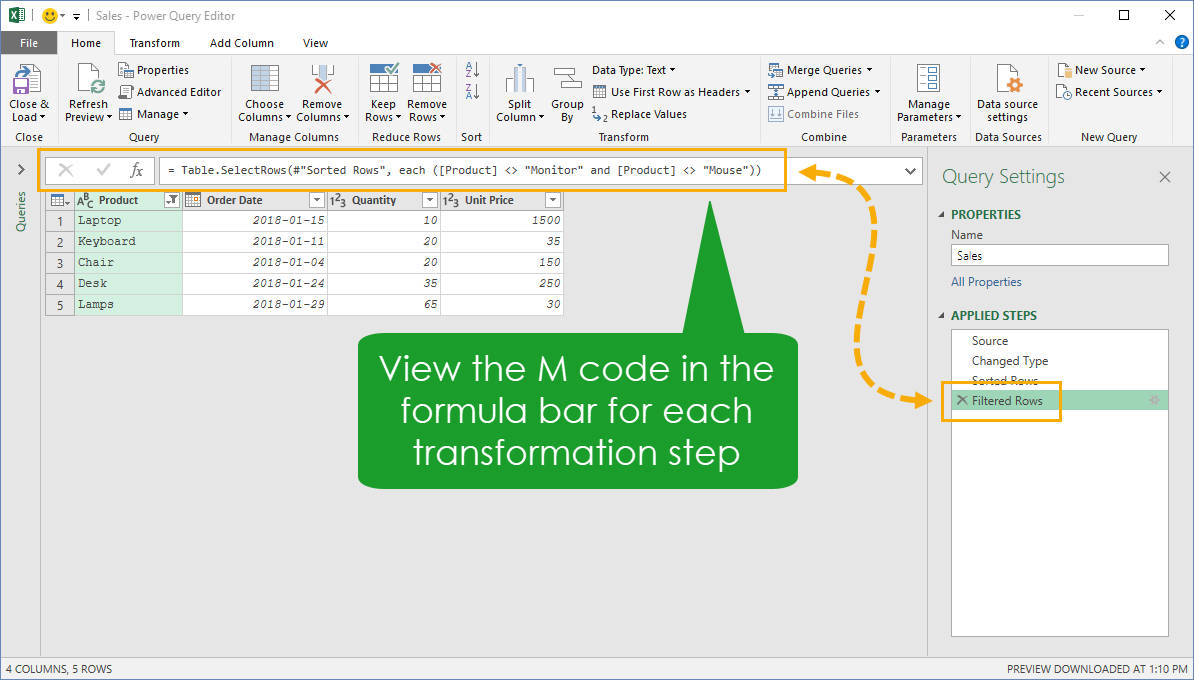For each step that’s created in the editor UI you can view the corresponding M code in the formula bar.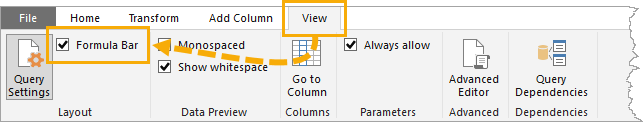If you don’t see the formula bar, go to the View tab and make sure the Formula Bar option is checked.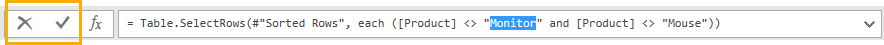You can edit the M code for any step in a query by clicking into the formula and editing the existing code. When finished, you can accept any changes by either clicking on the check mark or pressing Enter. You can also discard your changes by clicking on the X or pressing Esc.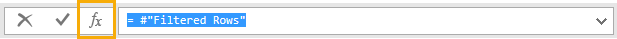You can also create entirely new steps in your query with the formula bar by clicking on the fx symbol next to the formula bar. This will create a new step that references the previous step by name and then you can create any M code you need to.

The formula bar only shows the M code for the currently selected step in the query, but the advanced editor is where you can view and edit the M code for the entire query.You can open the advanced editor from two places in the editor ribbon. From either the Home tab or the View tab press the Advanced Editor button.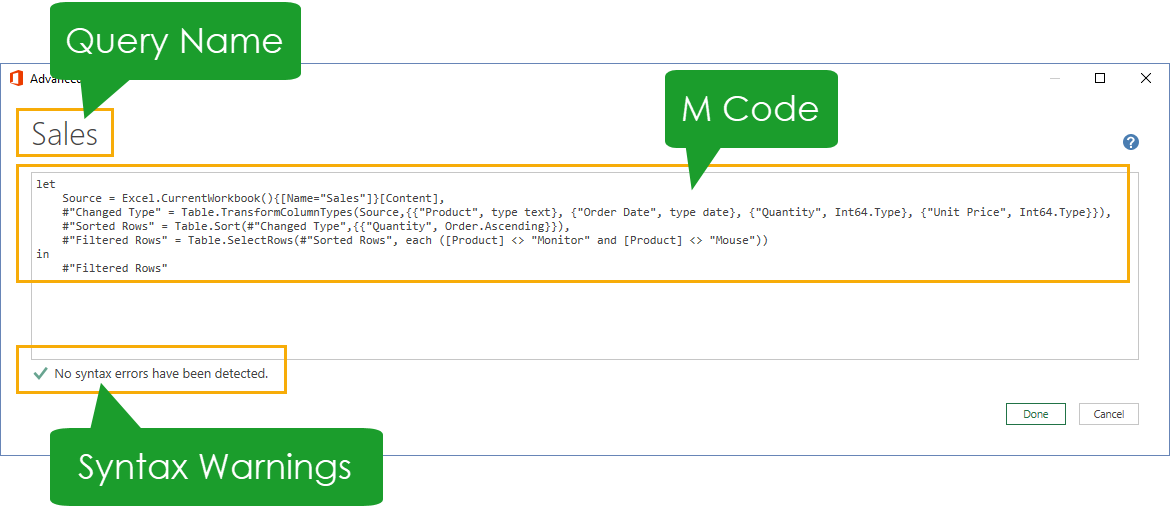Despite the “advanced” moniker, the editor is the most basic code editor you will see and doesn’t (yet) contain any intellisense auto complete, syntax highlighting or auto formatting features.

The advanced editor will display the query name, show the M code for the query and display a warning about any syntax violations in the M code. That’s it!

## Standard Function Library

Since M code is a functional language, it’s all about the functions and M code comes with a large library of predefined functions called the standard library.

Information on all the available standard library functions can be found on Microsoft’s Power Query M Reference web page, including function syntax and examples.

The standard library can also be explored from the power query editor using the #shared keyword.When entered into the formula bar, you can then explore all the available functions by clicking on the word Function to the right of the function’s name. You’ll find the same syntax and examples as the reference webpage.

## Case Sensitivity

One of the first thing someone needs to be aware of when writing M code is that it is a case sensitive language.

This means x is not the same thing as X or “abc” is not the same thing as “ABC”. This is true for any values, variables, functions etc.

## Expressions And Values In Power Query

Power query is all about Expressions and Values.

An expression is something that can be evaluated to return a value in power query. 1 + 1 is an expression that evaluates to the value 2.

A value is a single piece of data. Values can be single values such as numbers, text, logical, null, binary, date, time, datetime, datetimezone or durations.

Values can also have more complex structures than single values such as lists, records and tables. You can also have values that are a combination of lists, records and tables. Lists of lists, tables of lists, tables of tables etc… are all possible value structures.

### Single Literal Values

Single literal values are the basic building block of all the other values.

• 123.45 is a number value.
• “Hello World!” is a text value.
• true is a logical value.
• null represent the absence of a value.

### Single Intrinsic Values

Intrinsic values are constructed using the various intrinsic functions.

• #time(hours, minutes, seconds)
• #date(years, months, days)
• #datetime(years, months, days, hours, minutes, seconds)
• #datetimezone( years, months, days, hours, minutes, seconds, offset-hours, offset-minutes)
• #duration(days, hours, minutes, seconds)

For example, to construct the date 2018-12-31 you would need to construct it using the #date(2018, 12, 31) intrinsic function.

### Structured Values

#### ListsA List is an ordered sequence of values.

You can define a list using curly braces. {1, 2, 3} is a list containing the numbers 1, 2 and 3. Since the order is important, this is not the same list as {3, 2, 1}.

{“Hello”, “World”} is a list containing the text “Hello” and “World”.

Lists of lists are also possible, so {{1, 2}, {3, 4, 5}} is a list of two lists. The first list contains the number 1 and 2 and the second list contains the numbers 3, 4 and 5.

You can create sequential lists using the format {x..y}. {2..5} will produce the list {2, 3, 4, 5}. This also works for text characters too. {“a”..”d”} will produce the list {“a”, “b”, “c”, “d”}.

You can also have a list with no items, {} is the empty list.

Since lists are ordered, we can reference items in the list with a zero based index number. {1, 2, 3}{2} will evaluate to 3 since this is the 2nd item in the list (based on a zero index).

#### RecordsA Record is an ordered sequence of Fields. Each field consists of a field name which uniquely identifies the field and a field value which can be any type of value.

You can define a record using square braces. `[FirstName = "John", Age = 38]` is a record with two fields. The first field in the record has a field name of FirstName and value of “John”. The second field in the record has a field name of Age and a value of 38.

Records of records are also possible, `[Person = [FirstName = "John", Age = 38]]` is a record with one field with a field name of Person and a field value which is a record.

Empty records are also possible, [] is the empty record.

You can reference the field value in a record by its field name. [FirstName = “John”, Age = 38][FirstName] will evaluate to “John”.

#### TablesA Table is an ordered sequence of Rows where each row is a list.

Tables can only be constructed using an intrinsic function. You can construct a table using the #table() function from a list of column headings and a list of rows.

`#table({"Letters", "Numbers"}, {{"A", 1}, {"B", 2}, {"C", 3}})` will create a table with 2 columns, 3 rows and the column headings of Letters and Numbers.

It’s possible to create an empty table using empty lists in the #table() intrinsic function. #table({}, {}) will produce an empty table.

You can reference any value in a table with the zero based row index and the column heading name. `#table({"Letters", "Numbers"}, {{"A", 1}, {"B", 2}, {"C", 3}}){2}[Letters]` will evaluate to “C” since this is the 2nd row (based on a zero index) of the Letters column.

### Expressions

Expressions are anything that can be evaluated to a value. This is true of values themselves. For example, the expression 1 evaluates to the value 1.

Although you would typically think of expressions as being made up of more complex operations or functions.

For example:

• The expression 1 + 1 evaluates to 2.
• The expression 3 > 2 evaluates to true.
• The expression “Hello ” & “World” evaluates to “Hello World”.
• The expression Text.Upper(“Hello World”) evaluates to “HELLO WORLD”.

## Operators

Along with the standard library, M code also has a list of special functions called operators. These take exactly two values (or expressions that evaluate to a value) and return a single value.

### Arithmetic

M code comes with the basic arithmetic operators you would expect and are used to from regular Excel +, , * and /. These will allow you to add, subtract, multiply and divide values respectively.

These can be used with various other types of values other than just numbers. For example, you can add a duration to a date.

#date(2018,12,25) + #duration(7, 0, 0, 0) will evaluate to 2019-01-01.

### Comparison

You can compare values in M code using the comparison operators <, >, <=, >=, =, <>.

• x < y will evaluate to true if x is less than y.
• x > y will evaluate to true if x is greater than y.
• x <= y will evaluate to true if x is less than or equal to y.
• x >= y will evaluate to true if x is greater than or equal to y.
• x = y will evaluate to true if x is equal to y.
• x <> y will evaluate to true if x is not equal to y.

These can be used with various types of values. For example, you can compare two lists with the equal operator.

{1,2,3,4} = {1,2,3} will evaluate to false since the lists are not the same.

### Concatenation and Merger

You can concatenate text and merge lists, records and tables using the ampersand `&` operator.

For example:

`"Hello " & "World"` will evaluate to “Hello World”.

{1,2,3} & {3,4,5} will evaluate to {1,2,3,3,4,5}.

### Logical

You can perform operations on Boolean values (or expressions that evaluate to Boolean values) with the not, and and or operators.

## Commenting Code

There are two types of comments possible in M code. Single line comments and multi line comments.

``````M code goes here
M code goes here //This is a single line comment
M code goes here``````

A single line comment can be created by preceding the comment with two forward slash characters //. Anything on the same line before this will be interpreted as M code,  anything after it will be interpreted as a comment.

``````M code goes here /*This is a comment
on multiple lines*/ M code goes here``````

A multiple line comment can be created by placing the comment between /* and */ characters. Anything outside these will be interpreted as M code. Anything between these will be interpreted as a comment.

## Let Statement

The let statement allows a set of values to be evaluated and assigned to variable names then used in a subsequent expression that follows the in statement.

``````let
a = 1,
b = 2,
c = a + b
in
c``````

This expression is made up of three expressions that are evaluated after the let statement. Each expression is separated by a comma except for the last one before the in statement. In this example the entire let and in expression will evaluate to 3.

``````let
c = a + b,
b = 2,
a = 1
in
c``````

You might be thinking that the expressions within a let statement need to appear in the order they need to be evaluated. This is not the case! The above code is perfectly valid and will also evaluate to 3. The M code evaluator will automatically calculate the order of calculations needed based on expression dependencies.It’s obviously easier for a person to read the M code if it’s written in order of evaluation, but there is also another advantage. Expressions will appear as separate steps in the Applied Steps window. When written out of order, the expressions will appear as one combined step.

``````let
a = 1,
b = 2
in
a + b``````

You can also evaluate expressions within the in part of a let… in… expression.

## Variable Names

``````let
#"Is this a variable name? Wow!!!" = 1 + 1
in
#"Is this a variable name? Wow!!!"``````

You can assign just about any name to your expressions using the #”” characters. You can even use space characters and other special characters. Using reserved keywords is the only exception.The variable names are what will appear in the Applied Steps of the query editor, so being able to use space characters is a great feature.

## Each Statements

The each expression is a shorthand for declaring functions taking a single parameter named _ (underscore).

``````let
Source = #table({"Numbers"}, {{1}, {2}, {3}, {4}, {5}}),
in

In this example we are creating a new column which multiplies the Numbers column by 2 for each row.

``````let
Source = #table({"Numbers"}, {{1}, {2}, {3}, {4}, {5}}),
in

We can create the same query using the underscore syntax which is semantically equivalent to the each statement. Both queries will work the same.

## If Then Else Statements

M code is pretty sparse compared to other languages when it comes to logical expressions. There are no select case or loop statements available. There is only an if… then… else… expression available.

``````if [logical expression to test]
then [do this when true]
else [do this when false]``````

The syntax is straight forward and is like most other programming languages. It can appear all on one line, or it can be presented on separate lines for ease of reading.

## Try Otherwise StatementsErrors can happen when trying to perform operations that require particular types of data. For example trying to multiply a number with a text value will result in an error.

``````let
Source = #table({"Number", "Number and Text"}, {{2, 2}, {2, "Hello"}}),
#"Added Custom" = Table.AddColumn(Source, "Product", each try [Number]*[Number and Text] otherwise 0)
in

Errors can be avoided by using the try… otherwise… expression.This will avoid errors in your query results and allow you to replace errors with any value or expression.

## Functions

A function is a mapping from a set of parameter values to a value. Along with the functions in the standard library, M code allows you to create your own functions.

``````let
Product = (x,y) => x * y,
Result = Product(2,3)
in
Result``````

This query defines a function that multiplies two numbers. Then the query calls and evaluates the function with the values 2 and 3 which evaluates to 6.

### Functions With Optional Parameters

There are two types of function parameters, a required parameter and an optional parameter.

Required parameters must always be specified when a function is invoked.

Optional parameters do not need to be specified when a function is invoked. If the optional parameter is missing, then the value passed to the function will be null.

``````let
Product = (x, optional y) => if y is null then x else x * y,
Result = Product(2)
in
Result``````

This function has an optional second argument. Then the query calls and evaluates the function using only the required parameter with a value of 2, which evaluates to 2. Notice that the function needs to account for y being null, otherwise a missing optional argument could result in a function evaluating to an error.

### Recursive Functions

It is also possible to write a function that refers to itself by using the @ scoping operator.

``````let
Fibonacci = (n) =>
if n = 1
then 1
else
if n = 2
then 1
else @Fibonacci(n-1) + @Fibonacci(n-2),
Result = Fibonacci(7)
in
Result``````

The Fibonacci sequence is an example of a function that’s defined recursively. The next number in the sequence is defined as the sum of the two previous numbers. So to get the nth number, you need to know the (n-1)th and (n-2)th number.

This function will find the nth Fibonacci number by adding the (n-1)th and (n-2)th Fibonacci numbers.

The query evaluate to 13, since 13 is the 7th Fibonacci number.

Here are some more useful examples where you can use recursive functions to replicate Excel’s TRIM function to remove excess spaces between words or bulk find and replace values based on a list.

### Query Functions

The above examples defined a function within a query then called and evaluated the function within the query.

It is also possible to create a query which is a function (a query function) and can be called and evaluated from other queries.

``````let FunctionResult = (Argument1, Argument2,...) =>
let
/*M code to evaluate in the function goes here*/
in
Result
in
FunctionResult``````

This is the general format needed to create a query function. Note, you will need a let… in… statement within the let… in… statement of the query function in order to perform multiple steps.

## Conclusions

Power query is a great feature in Excel that can help you automate and simplify your data importing and transformation.

You can do a lot using just the graphical point and click interface without ever touching the M code. But as your requirements become more advanced, there might be a time where you need to edit the M code you’ve created or write your own from scratch.

Any new programming language is unreadable if you don’t know the basics first. This guide will hopefully get you up and running with M code so you can start creating more advanced queries.